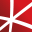# Top 20 NuGet algebra Packages

Math.NET Numerics is the numerical foundation of the Math.NET project, aiming to provide methods and algorithms for numerical computations in science, engineering and every day use. Supports .Net Framework 4.0 or higher and .Net Standard 1.3 or higher, on Windows, Linux and Mac.
Math.NET Numerics is the numerical foundation of the Math.NET project, aiming to provide methods and algorithms for numerical computations in science, engineering and every day use. Supports .Net Framework 4.0 or higher and .Net Standard 1.3 or higher, on Windows, Linux and Mac. This package contain...
F# Modules for Math.NET Numerics, the numerical foundation of the Math.NET project, aiming to provide methods and algorithms for numerical computations in science, engineering and every day use. Supports .Net Framework 4.5 or higher and .Net Standard 1.6 or higher, on Windows, Linux and Mac.
Math.NET Symbolics is a basic open source computer algebra library for .Net and Mono. Written in F# but works well in C# as well. Supports .Net Framework 4.5 or higher and .Net Standard 2.0 or higher, on Windows, Linux and Mac.
F# Modules for Math.NET Numerics, the numerical foundation of the Math.NET project, aiming to provide methods and algorithms for numerical computations in science, engineering and every day use. Supports .Net Framework 4.5 or higher and .Net Standard 1.6 or higher, on Windows, Linux and Mac. This pa...
Foundational classes for financial, engineering, and scientific applications, including complex number classes, general vector and matrix classes, structured sparse matrix classes and factorizations, general sparse matrix classes and factorizations, general matrix decompositions, least squares solut...
The GPU-accelerated version of package CenterSpace.NMath. With a few minor exceptions, such as optional GPU configuration settings, the API is identical between CenterSpace.NMath.Premium and CenterSpace.NMath. If using at least .NET Framework 4.6.1 or .NET Core 2.0, we recommend using one of our NMa...
Foundational classes for financial, engineering, and scientific applications, including complex number classes, general vector and matrix classes, structured sparse matrix classes and factorizations, general sparse matrix classes and factorizations, general matrix decompositions, least squares solut...
Foundational classes for financial, engineering, and scientific applications, including complex number classes, general vector and matrix classes, structured sparse matrix classes and factorizations, general sparse matrix classes and factorizations, general matrix decompositions, least squares solut...
The Extreme Optimization Numerical Libraries for .NET are a set of libraries for numerical computing and data analysis. This is the main package that contains all the core functionality. For optimal performance, we strongly recommend also referencing one of the native packages based on Intel's Mat...
Contains a matrix extension library, along with a suite of numerical matrix decomposition methods, numerical optimization algorithms for constrained and unconstrained problems, special functions and other tools for scientific applications. This package is part of the Accord.NET Framework.
Proviant is a framework which evaluate boolean-expressions under the help of the Shunting-yard algorithm.
Lightweight optimizer of System.Linq.Expression expressions. Just basic boolean algebra and reductions, constant and tuple/anonymous type eliminations. For side-effect free Expressions. No compilation-subjective optimizations.
Extreme Optimization Numerical Libraries for .NET Data Access Library for JSON.## NMath - Standard Library - OSX x64 (alpha)

Foundational classes for financial, engineering, and scientific applications, including complex number classes, general vector and matrix classes, structured sparse matrix classes and factorizations, general sparse matrix classes and factorizations, general matrix decompositions, least squares solut...
The Extreme Optimization Numerical Libraries for .NET are a set of libraries for numerical computing and data analysis. This package provides types specialized to single-precision, including complex numbers, as well as optimized implementations of single-precision linear algebra. Supports .NET Fra...
The Extreme Optimization Numerical Libraries for .NET are a set of libraries for numerical computing and data analysis. This package provides a complete implementation of linear algebra functionality for arbitrary numerical types. Supports .NET Framework 3.5, 4.0 and 4.6+, .NET Standard 1.3 and 2...
Bright Wire is an open source machine learning library. Includes neural networks (feed forward, convolutional and recurrent), naive bayes, linear regression, decision trees, logistic regression, k-means clustering and dimensionality reduction.## Bright Wire Machine Learning - GPU via CUDA Support

Bright Wire CUDA adds GPU support to Bright Wire. This lets you run Bright Wire machine learning on a Maxwell or better NVIDIA GPU.
The Extreme Optimization Numerical Libraries for .NET are a set of libraries for numerical computing and data analysis. This package contains the single-precision mixed-mode native provider. This is the recommended native provider for the classic .NET Framework on Windows. Supports .NET Framework ...